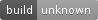## nightly fractran_macros

A compiler plugin that converts Fractran code into Rust at compile time, letting your Fractran programs be optimised by LLVM to super-fast native code

### 5 releases

Uses old Rust 2015

 0.1.5 Mar 21, 2015 Jan 26, 2015 Jan 4, 2015 Nov 20, 2014 Nov 14, 2014

#208 in Programming languages

MIT/Apache

15KB
260 lines

# `fractran_macros`A Rust macro for compiling FRACTRAN programs embedded in a Rust program into efficient, allocation-less, libcore-only1 code at compile time.

FRACTRAN is a very simple language; a program is an integer `n` along with a list of positive fractions, executed by finding the first fraction `f` for which `nf` is an integer, replace `n` by `nf` and repeating (execution halts when there is no such fraction). It turns out that this is Turing complete, and people have even written FRACTRAN interpreters in FRACTRAN! (See `examples/fractran.rs`.)

1That's right; you can now use FRACTRAN inside a kernel.

## Usage

The `fractran` macro takes a series of comma-separated arithmetic expressions, representing the sequence of fractions. Supported operations:

• `*`, `/` and parentheses for grouping; no risk of arithmetic overflow or loss of precision,
• `+`; can overflow,
• integer powers via `^`; no overflow, but precedence is incorrect, so `a^b * c` is `a^(b * c)`, rather than `(a^b) * c` as it should be. Use parentheses to ensure correctness.

The macro returns a constructor function `fn(&[u32]) -> T`, where `&[u32]` is the initial number (in the format described below), and `T` is a type implementing `Iterator<()>` and `fractran_support::Fractran`. Calling `next` will step the machine (i.e. finding the appropriate fraction as described above), returning `None` when the machine has halted.

The `fractran_support::Fractran` trait provides the `state` method (for viewing the current number, in exponent form) and the `run` method for executing the state machine until it halts.

### Example

``````#![feature(phase)]

#[phase(plugin)] extern crate fractran_macros;

fn main() {
// takes 2^a 3^b to 3^(a+b)
println!("{}", add(&[12, 34]).run()); // [0, 46]

// takes 2^a 3^b to 5^(ab)
let mult = fractran!(455 / 33, 11/13, 1/11, 3/7, 11/2, 1/3);
println!("{}", mult(&[12, 34]).run()); // [0, 0, 408, 0, ...]
}
``````

Remember to ensure the `Cargo.toml` has the appropriate dependencies:

``````[dependencies.fractran_macros]
git = "https://github.com/huonw/fractran_macros"
``````

## Representation

Numbers are represented in terms of the (32-bit) exponents of their prime factors. The `i`th entry of `[u32]` values is the exponent of the `i`th prime (zero-indexed), for example `2 == `, `3 == [0, 1]`, `9438 == 2 * 3 * 11^2 * 13 == [1, 1, 0, 0, 2, 1]`. This representation allows the implementation to handle very large numbers, anything where the largest exponent of any prime is less than 232, so the smallest non-representable number is 2232 = 24294967296.

This also allows the internal implementation to be highly efficient with just (statically determined) array indexing and integer comparisons & additions; there is no possibility of out-of-bounds indexing (and thus no performance penalty from unwinding), nor is there any division or remainder operations. As an example, the `example/prime.rs` program uses Conway's prime enumeration FRACTRAN program to generate primes, it takes only 6 seconds to do 1 billion steps (reaching the lofty heights of 887).

Converting this representation to and from an actual number can be achieved with your favourite prime-number crate (e.g. zipping with the primes iterator from `slow_primes`).

## Why?

Why not? Esolang-macros are fun, and so is the fundamental theorem of arithmetic.

~555KB
~11K SLoC